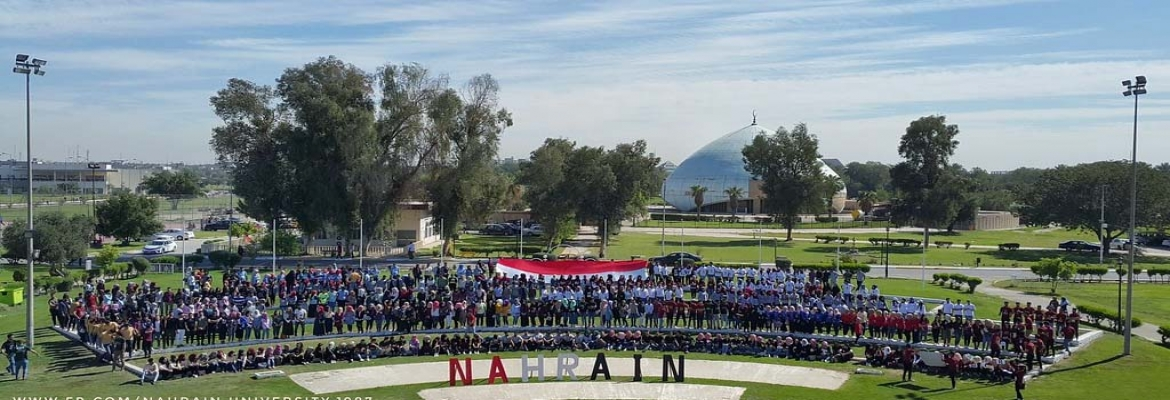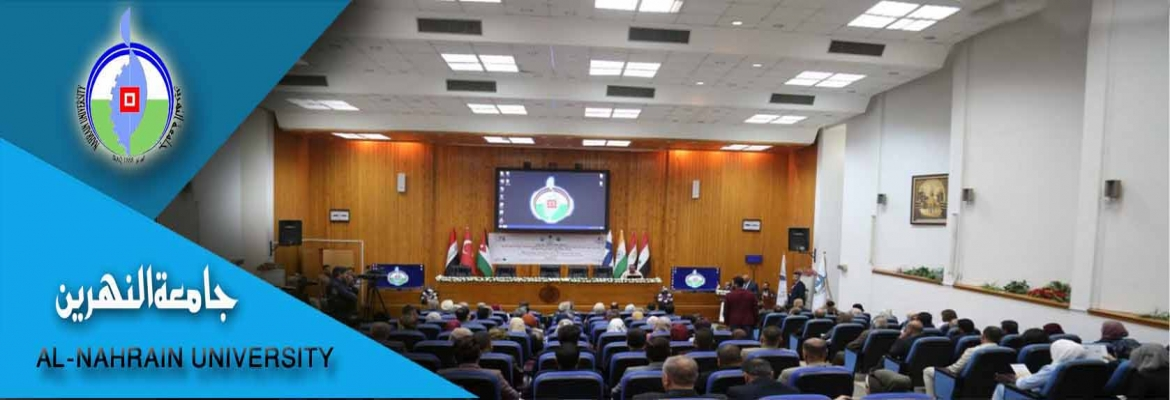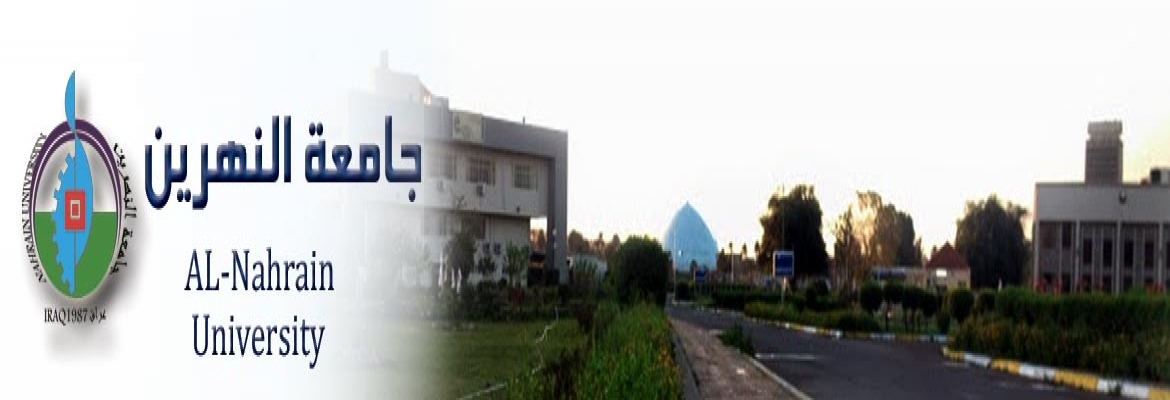# INVESTIGATION OF CORRELATION AND PREDICTION OF ENTHALPY OF PURE AND MIXTURE OF SATURATED VAPOR

number:
2028
Degree:
Author:
Supervisor:
Prof. Dr. Mahmoud Omar Abdullah,
year:
2009

The prediction of accurate values of saturated enthalpy of vapor is very important in process design calculations and other industrial applications. Experimental measurements of saturated enthalpy of vapor are not easy to perform. So in order to obtain accurate saturated enthalpy of vapor, attention has been turned to calculate them using equations of state. Some of these equations applied for both gas and liquid phases, and some of them applied for gas phase only, noting that those equations of state were developed primarily for calculating vapor-liquid equilibrium.To overcome these problems efforts were directed to modify or improve the equations of state. The accuracy of these equations are different depend on the selected type of systems. Five equations of state were used to calculate the residual enthalpy for pure compounds (polar and non polar). The AAD% for 25 pure compounds (polar and non polar) and 701 experimental data points are 2.5780, 6.7138, 1.8757, 2.2696, 3.4648, and 2.4914% when using Redlich-Kwong, Soave Redlich-Kwong, Peng-Robinson,Lee-Kesler, Virial equation truncated to second and to third terms respectively.It is considered from the results obtained above that PengRobinson equation is the best equation that can be used to calculate the residual enthalpy of the saturated vapor compared with the other equations of state mentioned.Efforts were therefore directed toward possibility of modifying Peng-Robinson equation of state to increase its accuracy in predicting the residual enthalpy of saturated vapor.For pure compounds,a new modified correlation was proposed to predict the enthalpy of saturated vapor. The modification was made by redefining the parameter as a function of reduced temperature,pressure and acentric factor by using statistical program.The correlation is given as in the following equationsThesis.pdf

## اخبار كلية الهندسة

Education - This is a contributing Drupal Theme
Design by WeebPal.